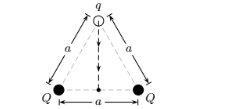Problem: The two charges Q are fixed at the vertices of an equilateral triangle with sides of length a as shown.The work required to move a charge q from the other vertex to the center of the line joining the fixed charges is1. W = √ 2kQq / a2. W = 03. W = 2kQq / a4. W = 4kQq / a5. W = kQq / a6. W = 6kQq / a

FREE Expert Solution
93% (454 ratings)
Problem Details

The two charges Q are fixed at the vertices of an equilateral triangle with sides of length a as shown.

The work required to move a charge q from the other vertex to the center of the line joining the fixed charges is

1. W = √ 2kQq / a

2. W = 0

3. W = 2kQq / a

4. W = 4kQq / a

5. W = kQq / a

6. W = 6kQq / a